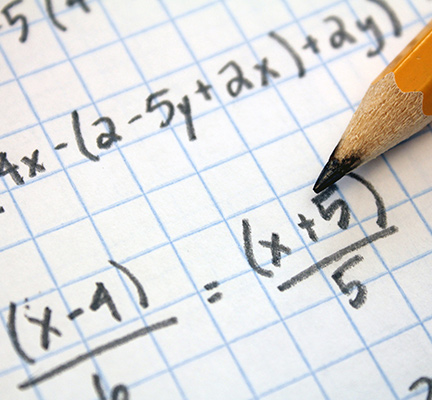Examination Board:  Edexcel

GCSE Mathematics 4360 helps students develop a knowledge and understanding of:

• problem solving
• reasoning
• applying maths in context
• the functional elements of maths.

This course is particularly suitable for students who wish to progress to A-level Mathematics.

### What will I study?

NUMBER

ALGEBRA

SHAPE

DATA

Algebraic notation

Angles on intersecting lines, in triangles and quadrilaterals, and on parallel lines

Data handling

Order numbers

Manipulate algebraic expressions

Interior and exterior angles of polygons

Bias

Factors, multiples and primes

Solve linear equations

Designing a survey

Squares, square roots, cubes and cube roots

Solve simultaneous equations with two unknowns

Reflection and rotation symmetry in 2D shapes

Design data collection methods

Index notation

Congruence and similarity

Tables and lists

Index laws

Using formulae

Pythagoras’ theorem

Two-way tables

Standard form

Solve linear inequalities

Trigonometry

Charts and diagrams

Equivalent fractions and adding and subtracting fractions

Trial and improvement

Parts of a circle

Types of average and range

Decimals, including recurring decimals

Sequences

Circle theorems and their proofs

Interpreting graphs and diagrams

Percentages

Nth term of a sequence

Using 2D diagrams to represent 3D shapes

Patterns in data

Using fractions, decimals and percentages

Coordinates

Transformations

Lines of best fit

Percentages and proportional change

Graphs

Straight edge and compass constructions

Comparing data

Direct and indirect proportion

Gradients of parallel and perpendicular lines

Loci

Using calculators

Fractions, decimals and percentages

Simultaneous equations (one linear and one quadratic)

Perimeter and area

Probability language and the probability scale

Number operations and the relationships between them, including order of operations and inverse operations

Other graphs

Area of a triangle

Estimates of probability and relative frequency

Ratio

Graphs of loci

Circumference and area of a circle

Listing events

Use surds and π in exact calculations

Graphs from quadratic and other functions

Volumes of prisms

Mutually exclusive outcomes

Rounding and approximation

Real life graphs

Complex shapes and solids

Independent events

Upper and lower bounds

Direct and inverse proportion

Vectors

Tree diagrams

Use a calculator effectively

Transformation of functions

Maps and scale drawings

Experimental data and theoretical probability

Enlargement of shapes, including solids

Interpretation and accuracy

Converting measurements

Estimation of measures

Bearings

Compound measures

Measure and draw lines and angles

Drawing using a ruler and protractor